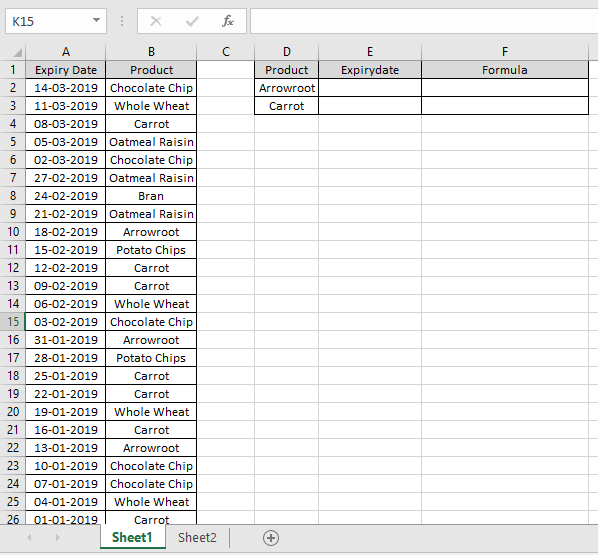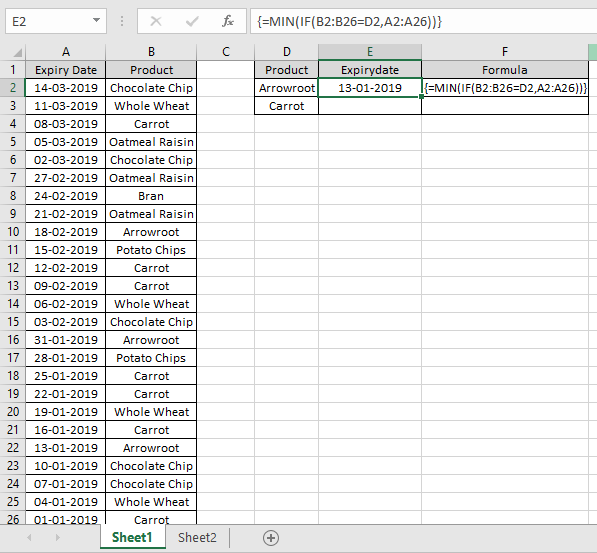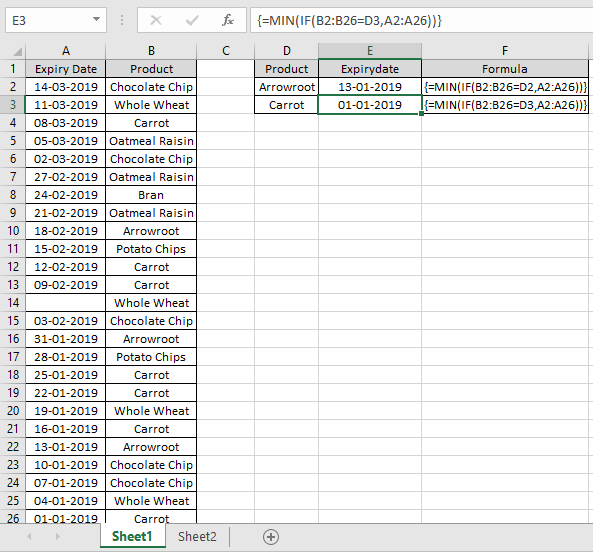# How to get the Minimum Value using IF function in excel

In this article, we will learn about how to get the minimum value if condition stands True in Excel.

In simple words, when working with long data sheets, sometimes we need to extract the minimum value if the condition stands True like finding the joining date by Employe code.

Let’s get the Generic Formula for this

{ =MIN (IF (range = criteria, values)) }

range = criteria : condition on the array
Values : corresponding array where minimum value to be find

Note:
Both array lengths must be equal
Donot put curly braces manually, USE Ctrl + Shift + Enter instead of just Enter after completion of formula.

Let’s understand this function using it an example.

Here we have expiry date of products and we need to find the earliest expiry date for the specific products.To get this right Excel MIN & IF functions will help.

We will use the formula for the product Arrowroot:

{ =MIN (IF (B2:B26 = D2, A2:A26))}

Explanation:
B2:B26 : range where formula matches the product name in D2 cell and returns an array of TRUE (1s) & FALSE (0s).
A2:A26 : Expiry date corresponding to range is multiplied

The formula drops down to
=MIN(IF( {0;0;0;0;0;0;0;0;TRUE;0;0;0;0;0;TRUE;0;0;0;0;0;TRUE;0;0;0;0}, {43538;43535;43532;43529;43526;43523;43520;43517;43514;43511;43508;43505;0;43499;43496;43493;43490;43487;43484;43481;43478;43475;43472;43469;43466}))

{0;0;0;0;0;0;0;0;43514;0;0;0;0;0;43496;0;0;0;0;0;43478;0;0;0;0}

This formula returns minimum value corresponding to all TRUE values

Convert the format of the cell to date using cell format option in Excel.13-01-2019 is the earliest expiry date for the arrowroot.

Use the formula for the product Carrot

{ =MIN (IF (B2:B26 = D3, A2:A26)) }As you can see This formula returns the earliest Expiry date (minimum value) for the specific product.

For version above Excel 2016 via OFFICE 365 user use Excel MINIFS function:

=MINIFS(values, range, criteria)

Hope you understood how to get the minimum value using IF function in Excel. Explore more articles on Excel logic functions here. Please feel free to state your query or feedback for the above article.

Related Articles:

How to use the SMALL function in Excel

How to Calculate Max if condition match in Excel

How to use the LARGE Function in Excel

How to use the MAX function in Excel

Retrieving the First Value in a List that is Greater / Smaller than a Specified Value in Excel

Terms and Conditions of use

The applications/code on this site are distributed as is and without warranties or liability. In no event shall the owner of the copyrights, or the authors of the applications/code be liable for any loss of profit, any problems or any damage resulting from the use or evaluation of the applications/code.